# TPD: Functional Diversity based on Trait Probability Density

#### 2019-07-02

This document illustrates the use of the package TPD for calculating several aspects of the functional diversity of biological communities. The functions included in TPD are based on the the trait probability densities of populations and species (TPDs), and its extension to communities (TPDc). These methods allow estimating several components of functional diversity and to partition it across scales. This collection of methods constitutes a unified framework that incorporates the underlying probabilistic nature of trait distributions in a uni- or multivariate trait space.

##The probabilistic nature of functional diversity

The collection of species that compose a community display a variety of trait values that conform a multivariate distribution of traits. As early as 1957, Hutchinson proposed his concept of niche, which is the multidimensional hypervolume in which a species can maintain a population. Hutchinsonian hypervolumes are generally conceived as uniform features, and all the points contained within them are assigned an equal probability of persistence of the species. Conseuquently, these hypervolumes can be characterised by their boundaries.

Nevertheless, this is not a totally satisfying conception, because some parts of the niche of a species present better conditions for subsistence than others. In correspondence, niches of species are usually described, conceived and represented as probability density functions. The same representation is generally applied to the trait distributions of species, reflecting the fact that individuals of a given species have some degree of variability in their trait values, and that some trait values (or combinations of trait values in the case of multiple traits) are more likely than others, just as it is more likely to find a 1.70 m tall person than a 2.10 m tall one.

Despite this, most methods analysing the functional diversity of communities are based on the consideration of a single value for each species and trait (the average values of each species for each trait), most species display non-negligible differences in the traits of their individuals. Probability density functions can be used to reflect the unequal probabilities of different trait values. Throughout this vignette, and in the TPD package, we will refer to these functions as Trait Probability Density (TPD).

Consider for instance the dataset `trees`, contained in the package `datasets` of R. It contains 31 observations of the girth, height and volume of black cherry trees. Let us calculate the TPD of the height for this population of cherry trees:

``````kde_height <- density(trees\$Height)
plot(kde_height, main = "TPD height")``````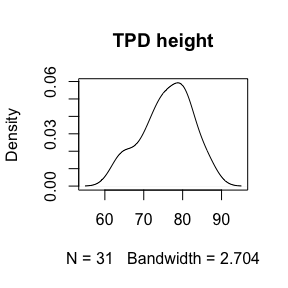The plot shows clearly that heights around 75 ft are much more likely than heights higher than 90 ft.

Because TPD’s are probability density functions, they integrate to 1, which means that the area under the plotted curve equals 1. To calculate this integral, we first expand a bit the range of traits of the database –we consider values between 50 and 100 ft; we want to be sure that our interval encompasses all the distribution–, and divide the trait axis into equal-length intervals. Although it is best to use a high number of intervals, in this example, for the sake of visibility, we have divided it into 50 intervals:

``````grid <- seq(from = 50, to = 100, length.out = 50)
edge_length <- grid - grid
#Each interval has this size (in trait units):
edge_length
#>  1.020408``````

Now, let us evaluate the TPD function in each point of the grid.

``````kde_height <- density(trees\$Height, from = 50, to = 100, n = 50)
plot(kde_height, main = "TPD height")
points(kde_height\$x, kde_height\$y, pch = 16, col = 2 , cex=0.6)
abline(v = grid, col = "grey50", lwd = 0.3)``````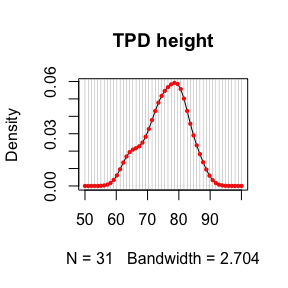The sum of the areas of all the rectangles with base `edge_length` = 1.02, and height equal to the value of the TPD function evaluated in each point of the grid is:

``````sum(edge_length * kde_height\$y)
#>  1.000979``````

Which is roughly 1! The TPD package uses kernel density estimations procedures to calculate the TPD of populations (when trait information of species is available at different locations, eg. different habitats) or species (when trait information of species is available at different points, and we assume a single TPD for the species regardless of location). We refer to these functions as TPDs. Because of the multidimensional nature of traits, the package allows estimating TPDs for single or multiple traits, with the function `TPDs`, which makes use of the kernel density estimation functions of the package ks. `TPDs` estimates a TPDs for each species or population included in the data.

##The `TPDs` function

To illustrate the use of `TPDs`, we are going to use the database `iris`, which contains measurements (in cm) of the sepal length and width and petal length and width, for 50 flowers from each of 3 species of Iris (I. setosa, I. versicolor, and I. virginica)

``````head(iris)
#>   Sepal.Length Sepal.Width Petal.Length Petal.Width Species
#> 1          5.1         3.5          1.4         0.2  setosa
#> 2          4.9         3.0          1.4         0.2  setosa
#> 3          4.7         3.2          1.3         0.2  setosa
#> 4          4.6         3.1          1.5         0.2  setosa
#> 5          5.0         3.6          1.4         0.2  setosa
#> 6          5.4         3.9          1.7         0.4  setosa``````

Suposse that we want to calculate the TPDs of each species considering `Sepal.Length` and `Sepal.Width`. Estimating these TPDs’s is very simple:

``````traits_iris <- iris[, c("Sepal.Length", "Sepal.Width")]
sp_iris <- iris\$Species
TPDs_iris <- TPDs(species = sp_iris, traits_iris)
#> -------Calculating densities for One population_Multiple species-----------``````

After a few seconds of calculations, the object `TPDs_iris` is created. This object, of class TPDsp contains, along with other information, the grid of cells in which the function was evaluated, contained in `TPDs_iris\$data\$evaluation_grid`, and the probability associated to each of those cells, contained in a list `TPDs_iris\$TPDs` with one element for each species:

``````head(TPDs_iris\$data\$evaluation_grid)
#>   Sepal.Length Sepal.Width
#> 1     3.760000        1.64
#> 2     3.783518        1.64
#> 3     3.807035        1.64
#> 4     3.830553        1.64
#> 5     3.854070        1.64
#> 6     3.877588        1.64
nrow(TPDs_iris\$data\$evaluation_grid)
#>  40000
names(TPDs_iris\$TPDs)
#>  "setosa"     "versicolor" "virginica"
#>        22455        22255        22655        22054        22254
#> 0.0006073417 0.0006067314 0.0006064457 0.0006051767 0.0006050836
#>        22055
#> 0.0006045352
sum(TPDs_iris\$TPDs\$setosa)
#>  1``````

There are a lot of cells in the grid, resulting from dividing each axis into 200 equal intervals. This number could be modified with the argument `n_divisions` of `TPDs`, which defaults to 200 for 2 dimensions, but increasing it results in higher computation times (because the number of cells increases exponentially with dimensions), with rather insignificant improvements in accuracy. Most importantly, `TPDs_iris` contains the TPDs of the three species (in `TPDs\$TPDs`), which are vectors containing the probability associated to each point of the evaluation grid:

``````summary(TPDs_iris\$TPDs)
#>            Length Class  Mode
#> setosa     40000  -none- numeric
#> versicolor 40000  -none- numeric
#> virginica  40000  -none- numeric
# The sum of all the elements in each TPDs is equal to 1:
sapply(TPDs_iris\$TPDs, sum)
#>     setosa versicolor  virginica
#>          1          1          1``````

The function `plotTPD` can be used to visualize the resulting TPDs’s. Note that it can only handle 1 or 2-dimensional data, and that in the case of 2 dimensional plots, the packages gridExtra and ggplot2 must be installed.

``````plotTPD(TPD = TPDs_iris, nRowCol = c(1,3))
#> Be patient, in the 2-dimensional case, plots can take some time.
#> If you think it takes too long, consider reducing the number of plots using 'whichPlot'``````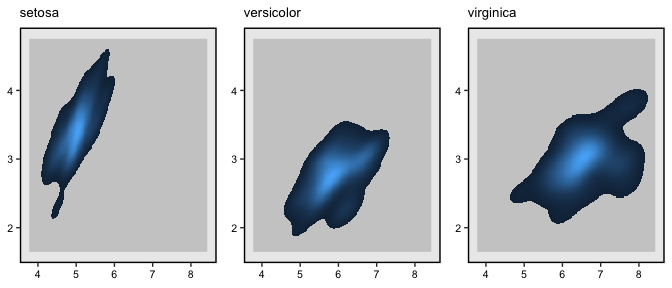Now that we are able to visualize TPD’s, we are going to show an important aspect of their construction: alpha. The parameter `alpha` determines the proportion of the probability density function of each species or population that will be included in the resulting TPD (Blonder et al. 20114; Swanson et al. 2015). This means that setting `alpha = 1` will include the whole function, whereas `alpha = 0.5` will include only a 50%. It is important to remarks that in the cases in which `alpha` is set to a value smaller than 1, the `TPD` function rescales the probabilities so that they add up to 1. By default, `alpha` is set to 0.95; thus `TPDs` is not too sensitive to outliers. Let us visualize the effect of alpha in the calculation of a 1-dimensional TPD. First, lets set `alpha = 1`:

``````traits_iris_d1 <- iris[, c("Sepal.Length")]
sp_iris <- iris\$Species
TPDs_iris_d1_a1 <- TPDs(species = sp_iris, traits = traits_iris_d1, alpha=1)
#> -------Calculating densities for One population_Multiple species-----------
plotTPD(TPD = TPDs_iris_d1_a1, nRowCol = c(1,3)) ``````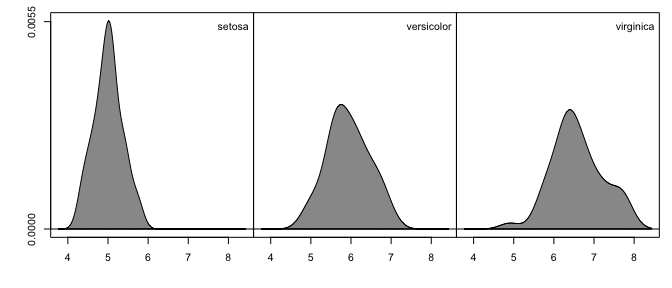Now, `alpha = 0.95`, the default value:

``````TPDs_iris_d1_a095 <- TPDs(species = sp_iris, traits = traits_iris_d1, alpha=0.95)
#> -------Calculating densities for One population_Multiple species-----------
plotTPD(TPD = TPDs_iris_d1_a095, nRowCol = c(1,3)) ``````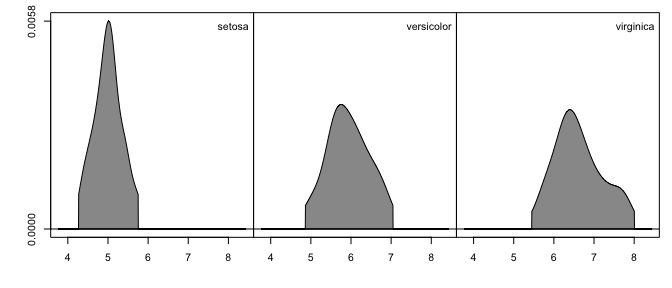And this is how `alpha = 0.5` looks like:

``````TPDs_iris_d1_a05 <- TPDs(species = sp_iris, traits = traits_iris_d1, alpha=0.5)
#> -------Calculating densities for One population_Multiple species-----------
plotTPD(TPD = TPDs_iris_d1_a05, nRowCol = c(1,3)) ``````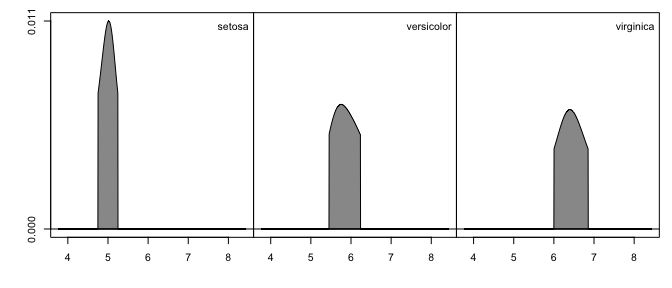Indeed, the same logic applies for higher dimensions:

``````TPDs_iris_a95 <- TPDs(species = sp_iris, traits = traits_iris, alpha=0.95)
#> -------Calculating densities for One population_Multiple species-----------
plotTPD(TPD = TPDs_iris_a95, nRowCol = c(1,3))
#> Be patient, in the 2-dimensional case, plots can take some time.
#> If you think it takes too long, consider reducing the number of plots using 'whichPlot'````````````TPDs_iris_a75 <- TPDs(species = sp_iris, traits = traits_iris, alpha=0.75)
#> -------Calculating densities for One population_Multiple species-----------
plotTPD(TPD = TPDs_iris_a75, nRowCol = c(1,3))
#> Be patient, in the 2-dimensional case, plots can take some time.
#> If you think it takes too long, consider reducing the number of plots using 'whichPlot'``````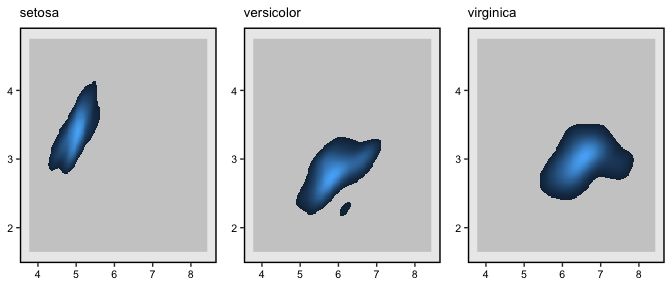(NOTE: Be careful when making 2 dimensional plots. If you try to plot too many species or populations at the same time, it can take quite a long time. We recommend to use the argument `whichPlot` in `PlotTPD` to control for this.)

As we mentioned above, sometimes there is trait information at the popolation level. Imagine that we have measured traits on individuals of each species in two different environments. The argument `samples` of `TPDs` allows us to calculate a separate TPDs for each population of each species. For example, suppose that the first 25 observation of each species belong to “Site.1” and the other 25 belong to “Site.2”.

``````pops_iris <- rep(c(rep("Site.1", 25), rep("Site.2", 25)), 3)
TPDs_pops_iris <- TPDs (species = sp_iris, traits = traits_iris, samples = pops_iris)
#> -------Calculating densities for Multiple populations_Multiple species-----------
plotTPD(TPD = TPDs_pops_iris, nRowCol = c(3,2))
#> Be patient, in the 2-dimensional case, plots can take some time.
#> If you think it takes too long, consider reducing the number of plots using 'whichPlot'``````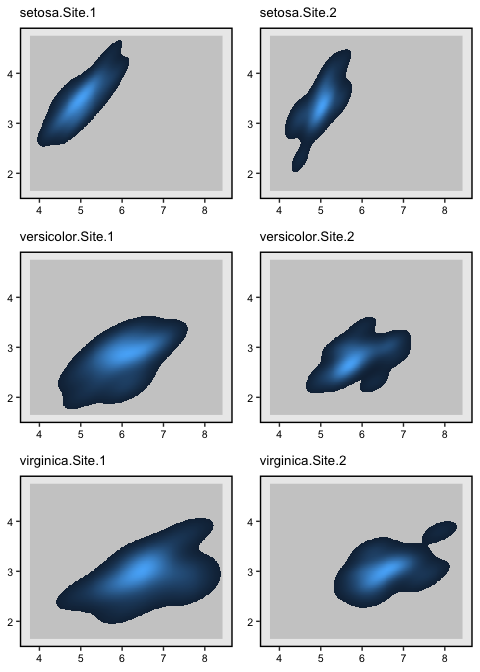Because the assignment of individuals to sites in this example is somewhat random, there are not important differences in the TPDs of populations of the same species. However, in real conditions, differences in the functional traits between conspecifics living under different conditions can be substantial, and therefore it is important to take this aspect into account (Carmona et al. 2015).

The `TPDs` function requires a high quantity of information to work: one measurement per individual and trait cosidered, and all the traits must be measured in all individuals in order to place them in the multidimensional space. In addition, several individuals are required to estimate reliable TPDs’s. In many occasions, we do not have access to such a high-quality information. The `TPDsMean` function is designed for those cases. `TPDsMean` performs the same task as `TPDs`, but it estimates the probabilities by calculating a multivariate normal distribution for each species or population, rather than using kernel density estimations. Hence, the only information required is the mean and standard deviation of each trait considered for each species or population. Let us imagine that we only have the means and standard deviations of the iris species:

``````names_iris <- unique(iris\$Species)
mt1 <- tapply(iris[, "Sepal.Length"], iris\$Species, mean)
mt2 <- tapply(iris[, "Sepal.Width"], iris\$Species, mean)
means_iris <- matrix(c(mt1, mt2), ncol=2)
st1 <- tapply(iris[, "Sepal.Length"], iris\$Species, sd)
st2 <- tapply(iris[, "Sepal.Width"], iris\$Species, sd)
sds_iris <- matrix(c(st1, st2), ncol=2)
TPDsMean_iris<- TPDsMean(species = names_iris, means = means_iris, sds = sds_iris)
#> -------Calculating densities for One population_Multiple species-----------
plotTPD(TPD = TPDsMean_iris, nRowCol = c(1,3))
#> Be patient, in the 2-dimensional case, plots can take some time.
#> If you think it takes too long, consider reducing the number of plots using 'whichPlot'``````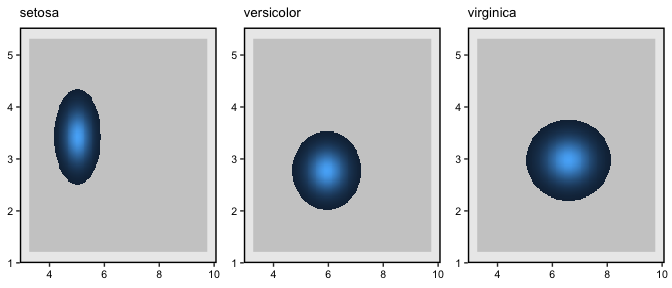## `TPDc`: from species to communities

One interesting feature of TPD’s is that the concept presented above for species or pupulations can be easily scaled up to express the functional structure of communities. This is the goal of the function `TPDc`. `TPDc` combines the information contained in the TPDs of the species in the community with information about their abundances, resulting in the Trait Probabiity Density of the community (which we called TPDc). The rationales is very simple: for each cell of the grid in which the functional space is divided, TPDc is the result of summing the probability of each of the species present in the community,weighted by their relative abundances.

We can illustrate this concept with the `iris` example. Let us imagine three different communities containing different abundances of each species:

``````abundances_comm_iris <- matrix(c(c(0.5, 0.4, 0), #I. virginica absent
c(0.0, 0.9,  0.1 ), #I. versic. dominates; setosa absent
c(0.0, 0.1,  0.9 )), #I. virg. dominates; setosa absent
ncol = 3, byrow = TRUE, dimnames = list(paste0("Comm.",1:3),
unique(iris\$Species))) ``````

To calculate the TPDc’s of a group of communities, we first have to calculate the TPDs of all the species present in each community. (This step is important: if you do not have the TPDs of all the species or populations, you cannot estimate the TPDc of the community, and `TPDc` will end with an error).

``````TPDs_iris <- TPDs(species = sp_iris, traits_iris)
#> -------Calculating densities for One population_Multiple species-----------
TPDc_iris <- TPDc(TPDs = TPDs_iris, sampUnit = abundances_comm_iris )``````

The resulting object (`TPDc_iris`) is an object of class TPDcomm, containing several pieces of information inherited from `TPDs_iris`, such as the coordinates of the evaluation grid (in `TPDc_iris\$data\$evaluation_grid`).

What is new in this object is the information contained in its component `\$TPDc`. Specifically, we are interested in the element `TPDc_iris\$TPDc\$TPDc`, which is a list with an element for each community (`length(TPDc_iris\$TPDc\$TPDc) =` 3), each one containing the probability associated to each cell of the grid in each community. In fact, the TPDc of a community reflects the probability of observing a given trait value when extracting a random individual from the community. Because TPDc’s are probability density functions, they integrate to 1:

``````sapply(TPDc_iris\$TPDc\$TPDc, sum)
#> Comm.1 Comm.2 Comm.3
#>      1      1      1``````

(NOTE: the probabilities contained in a TPDc will always sum 1, regardless of the values of abundance provided to the `TPDc` function, which are automatically transformed to relative abundances)

Similar to what we did with TPDs’s, `plotTPD` can be used to provide a graphical representation of TPDc’s of 1 or 2 dimensions. Let us examine the three communities:

``````plotTPD(TPD = TPDc_iris, nRowCol = c(1,3))
#> Be patient, in the 2-dimensional case, plots can take some time.
#> If you think it takes too long, consider reducing the number of plots using 'whichPlot'``````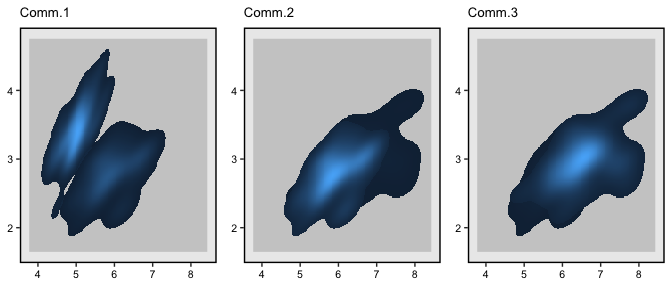The plots clearly reflect our expectations when we created the communities: the part of the functional space occupied by I. setosa is empty in Comm.2 and Comm.3, where that species is not present. In addition, Comm.2 and Comm.3, which share the same two species (I. versicolor and I. virginica), occupy the same part of the functional space. However, because of the different abundances of their species, the TPDc’s of these communities differ, reflecting the higher abundance of I. versicolor in Comm. 2 and of I. virginica in Comm.1.

## A unified framework for functional diversity

TPDs’s and TPDc’s are powerful tools for studying the functional diversity of biological communities. The TPD package includes several functions that estimate different indices reflecting complimentary aspects of functional diversity. With the aim to help users to select for the most adequate method depending on specific questions, we divide these methods into four different categories attending to two independent criteria.

The first criteria is whether the identity or number of species is or not of primary concern. In some cases (1), we are only interested on the aggregated probability distribution, that is, the TPD of the studied entity (which can be species, populations or communities). For example, if we are interested on how does the ammount of functional volume occupied by communities vary across environmental gradients, we only need to know the TPDc’s of communities. In such case, it would have been correct to sample and measure traits from random individuals in each community, constructing the TPDC’s without having identified any species. By contrast (2), sometimes the interest is not only on the TPD of the community, but on how the different species that compose it are organised in the functional space. This includes the study of functional diversity in terms of the functional dissimilarity of species, or the study of functional redundancy.

These two groups can be further divided according to whether (a) we are interested in studying properties only at the within-unit level (units can be species, populations or communities), or (b) we are interested in analysing the differences between these units, for which we have to consider more than one unit simultaneously.

The next table shows the methods that compose the framework, classified according to the above-stated criteria, along with the associated specific functions included in the TPD package:

(1) Interest is only on the aggregated probability distribution (2) Interest is not only on the aggregated probability distribution
(a) Within units Functional Richness, Evenness and Divergence (`REND`) Functional redundancy (`redundancy`)
Traits sampling (`tSamp`) Species dissimilarity (`dissim`)
(b) Between units Overlap-based dissimilarity (`dissim`) Partition of functional diversity with Rao (`dissim` + `Rao`)
Dissimilarity decomposition (`dissim`)

## 1. Species are not of primary concern

### 1.a. Indices for single units

The methods included in this category are intended for cases in which we are purely interested in some feature of the functional structure of individual populations, species or communities. This means that the results yielded for each unit of analysis is totally independent from the rest of units. The methods included here, and the associated functions are:

####Functional Richness, Evenness and Divergence (`REND`)

These three indices of functional diversity were originally described by Mason et al. (2005). Because a deeper study of their biological significance is out of the scope of this document, we refer readers to that reference for further details.

Functional richness (FRic) is the amount of functional volume occupied by the analysed unit (species/population/community). Therefore, a species with great variability in traits between their individuals will display a higher FRic value than a species with less variability.

Functional evenness (FEve) reflects the evenness of the abundance of the different trait values in the analysed unit. Imagine two communities with the same functional richness (the same degree of variability in traits between their individuals). Suppose that some traits are much more abundant than others in the first species, whereas all the traits have a similar abundance in the second species: FEve for the second species should be higher than FEve for the first one.

Functional divergence (FDiv) reflects the distribution of abundances within the functional trait volume occupied by the analysed unit. A species in which the most abundant trait values are away from the center of gravity of the functional space –eg. individuals are either very small, or very big– will display high FDiv, whereas a species in which the most abundant trait values are similar –eg. most individuals having intermediate size– will have low FDiv .

The function `REND` calculates these three indices using the TPDs’s and TPDc’s of populations/species and communities, respectively. The function works with one or more dimensions, thus expanding the framework presented in Mason et al. (2005) to multiple traits without modifying its original rationale, based on probabilities. Let us start with species with one and two dimensions:

``````TPDs_iris_d1 <- TPDs(species = sp_iris, traits_iris_d1)
#> -------Calculating densities for One population_Multiple species-----------
RED_d1 <- REND(TPDs = TPDs_iris_d1)
#> Calculating FRichness of species
#> Calculating FEvenness of species
#> Calculating FDivergence of species

TPDs_iris_d2 <- TPDs(species = sp_iris, traits_iris)
#> -------Calculating densities for One population_Multiple species-----------
RED_d2 <- REND(TPDs = TPDs_iris_d2)
#> Calculating FRichness of species
#> Calculating FEvenness of species
#> Calculating FDivergence of species``````

`RED_d1` and `RED_d2` contain the FRic, FEve and FDiv values of the three species considering one and 2 traits simultaneously. We can plot the respective TPDs’s, along with their FRich values:

``````plotTPD(TPD = TPDs_iris_d1, nRowCol = c(1, 3), leg.text = paste0(names(RED_d1\$species\$FRichnes),
"; FRic=", round(RED_d1\$species\$FRichnes, 3)))``````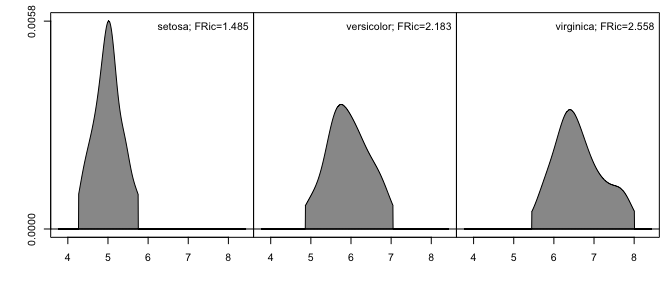``````plotTPD(TPD = TPDs_iris_d2, nRowCol = c(1, 3), leg.text = paste0(names(RED_d2\$species\$FRichnes),
"; FRic=", round(RED_d2\$species\$FRichnes, 3)))
#> Be patient, in the 2-dimensional case, plots can take some time.
#> If you think it takes too long, consider reducing the number of plots using 'whichPlot'``````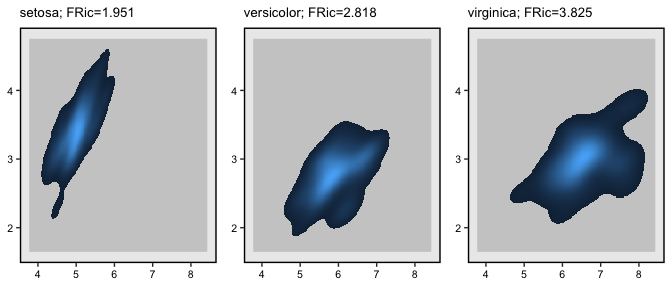The first thing to underscore is that FRic is given in the original trait units, which means that in the one-dimensional case (`Sepal.Length`), FRic is expressed in cm and in the two dimensional case (`Sepal.Length` and `Sepal.Width`) it is expressed in cm2. I. virginica is the species that occupies the biggest proportion of the functional space, both for one and two traits, and I. setosa the one occupying the smallest proportion.

The plots also reveal that I. setosa occupies a portion of the functional space that is distinct from the ones occupied by the other two species, which are more similar –with I. versicolor occupying a slightly more central position than I. virginica. We are going to take advantage of this observation to show the calculation of FDiv, and its application to communities. Let us create three communities, with different abundances of each species:

``````abundances_comm_iris_divergence <- matrix(c(c(0.45, 0.1, 0.45), # Different species are more abundant
c(0.05, 0.9,  0.05 ),  # Different species are less abundant
c(0.9,  0.05, 0.05 )), # A species with extreme trait values is very abundant
ncol = 3, byrow = TRUE, dimnames = list(paste0("Comm.",1:3),
unique(iris\$Species)))

TPDc_iris_divergence_d1 <- TPDc(TPDs = TPDs_iris_d1, sampUnit = abundances_comm_iris_divergence)
RED_comm_d1 <- REND(TPDc = TPDc_iris_divergence_d1)
#> Calculating FRichness of communities
#> Calculating FEvenness of communities
#> Calculating FDivergence of communities
plotTPD(TPD = TPDc_iris_divergence_d1, nRowCol = c(1, 3),
leg.text = paste0(names(RED_comm_d1\$communities\$FDivergence),
"; FDiv=", round(RED_comm_d1\$communities\$FDivergence, 3)))``````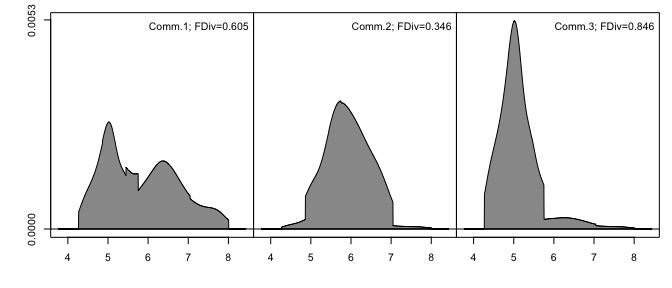``````
TPDc_iris_divergence_d2 <- TPDc(TPDs = TPDs_iris_d2, sampUnit = abundances_comm_iris_divergence)
RED_comm_d2 <- REND(TPDc = TPDc_iris_divergence_d2)
#> Calculating FRichness of communities
#> Calculating FEvenness of communities
#> Calculating FDivergence of communities
plotTPD(TPD = TPDc_iris_divergence_d2, nRowCol = c(1, 3),
leg.text = paste0(names(RED_comm_d2\$communities\$FDivergence),
"; FDiv=", round(RED_comm_d2\$communities\$FDivergence, 3)))
#> Be patient, in the 2-dimensional case, plots can take some time.
#> If you think it takes too long, consider reducing the number of plots using 'whichPlot'``````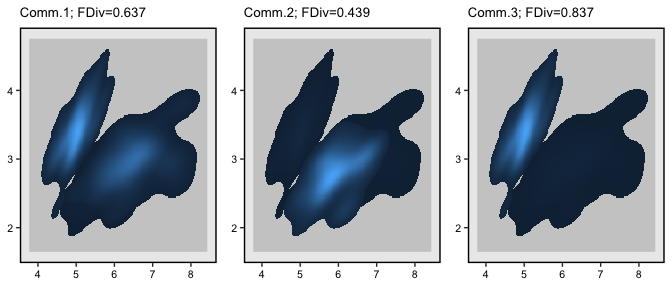Comm.3, with a great proportion of their TPDc being away from the center, displays the highest FDiv value, followed by Comm.1. By contrast, Comm.2, where I. versicolor dominates, has the lowest FDiv value. Interestingly, given that the three communities are composed of the same species, they have the same value of FRic, which is independent of species abundances:

``````RED_comm_d1\$communities\$FRichness
#>   Comm.1   Comm.2   Comm.3
#> 3.738378 3.738378 3.738378
RED_comm_d2\$communities\$FRichness
#>   Comm.1   Comm.2   Comm.3
#> 6.331625 6.331625 6.331625``````

To show the use of functional evenness, we are going to create an artificial set composed of two groups of four species each. Species in each group have the same mean trait value, but different variances. For the sake of visibility, we are going to present a one-dimensional example, but the same applies for higher dimensions.

``````set.seed(1)
sp_means <- rep(seq(0, 8, length.out = 4), 2)
sp_sds <- rep(c(0.5, 1), each=4)
sp_trait <- numeric()
n_indiv <- 50
for (i in 1:length(sp_means)) {
sp_trait <- c(sp_trait, rnorm(n_indiv, sp_means[i], sp_sds[i]))
}
sp_names <- rep(paste0("SP.", 1:length(sp_means)), each=n_indiv)
TPDs_sp <- TPDs(species = sp_names, traits = sp_trait, alpha = 0.99)
#> -------Calculating densities for One population_Multiple species-----------
plotTPD(TPD = TPDs_sp, nRowCol = c(2,4))``````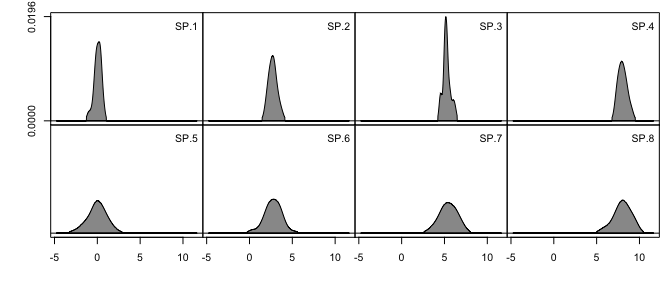Now, lets create four communities, two with the species in the first group, and two with the species in the second group, and examine their FEve values:

``````
comm_abundances <- matrix(c( c(0.25, 0.25, 0.25, 0.25, 0,    0,    0,    0), #Comm1, goup 1, equal abundances
c(0,    0,    0,    0,    0.25, 0.25, 0.25, 0.25), #Comm2, goup 2, equal abundances
c(0.85, 0.05, 0.05, 0.05, 0,    0,    0,    0), #Comm3, goup 1, unequal abundances
c(0,    0,    0,    0,    0.85, 0.05, 0.05, 0.05)), #Comm4, goup 2, unequal abundances
ncol = 8, byrow = TRUE, dimnames = list(paste0("Comm.",1:4),
unique(sp_names)))

TPDc_sp <- TPDc(TPDs = TPDs_sp, sampUnit = comm_abundances)
RED_sp <- REND(TPDc_sp)
#> Calculating FRichness of communities
#> Calculating FEvenness of communities
#> Calculating FDivergence of communities
plotTPD(TPDc_sp, nRowCol = c(2,2), leg.text = paste0(names(RED_sp\$communities\$FEvenness),
"; FEve=", round(RED_sp\$communities\$FEvenness, 3)))``````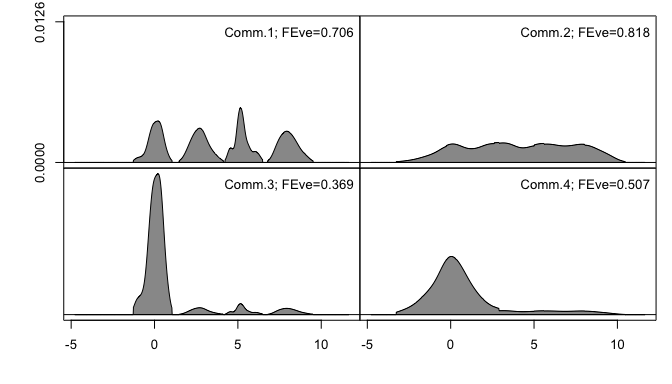The difference between Comm.1 and Comm.2 reflecs the effect of the evenness in the distribution of probabilities within the species that compose the communities. The probabilities associated to the trait values are generally more even in the species of Comm2 than in those of Comm1, hence the higher value of FEve (0.818 vs 0.706). Comm.3 and Comm.4 are composed of the same species than Comm1. and Comm.2, but with uneven relative abundances, thus reflecting the effect of the evenness in the abundance of species.

####Traits sampling (`tSamp`)

Some applications based on the traits of species require simulating trait values that could be found in a given population, species or community. The function `tSamp` performs this task. Lets say that we want to simulate 1,000 trait values from each Iris species:

``Iris_samp <- tSamp(TPDs=TPDs_iris, size=1000)``

`Iris_samp` contains three groups of 1,000 simulated trait values, one for each species. As usual, visualizing the results can be useful:

``````plotTPD(TPDs_iris, nRowCol = c(1,3))
#> Be patient, in the 2-dimensional case, plots can take some time.
#> If you think it takes too long, consider reducing the number of plots using 'whichPlot'````````````par(mfrow=c(1,3))
for (i in 1:3){
plot(Iris_samp\$species_samples[[i]], pch = 16, cex = 0.6,
xlim = range(TPDs_iris\$data\$evaluation_grid[]),
ylim = range(TPDs_iris\$data\$evaluation_grid[]))
}``````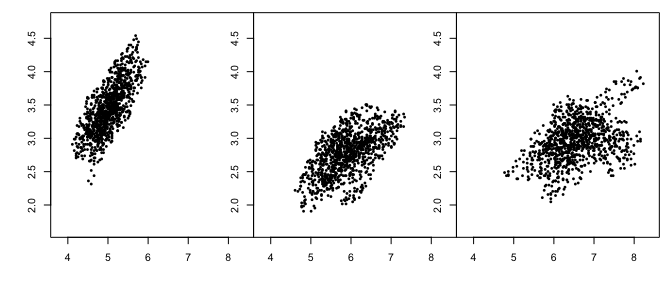Indeed, `tSamp` also works with TPDc’s, simulating trait values that could have been drawn from a community. We can recover the object `TPDc_iris` that we created above, draw 1,000 trait values from each community, and plot them:

``````Iris_comm_samp <- tSamp(TPDc = TPDc_iris, size = 1000)
plotTPD(TPDc_iris, nRowCol = c(1,3))
#> Be patient, in the 2-dimensional case, plots can take some time.
#> If you think it takes too long, consider reducing the number of plots using 'whichPlot'````````````par(mfrow=c(1,3))
for (i in 1:3){
plot(Iris_comm_samp\$communities_samples[[i]], pch = 16, cex = 0.6,
xlim = range(TPDc_iris\$data\$evaluation_grid[]),
ylim = range(TPDc_iris\$data\$evaluation_grid[]))
}``````